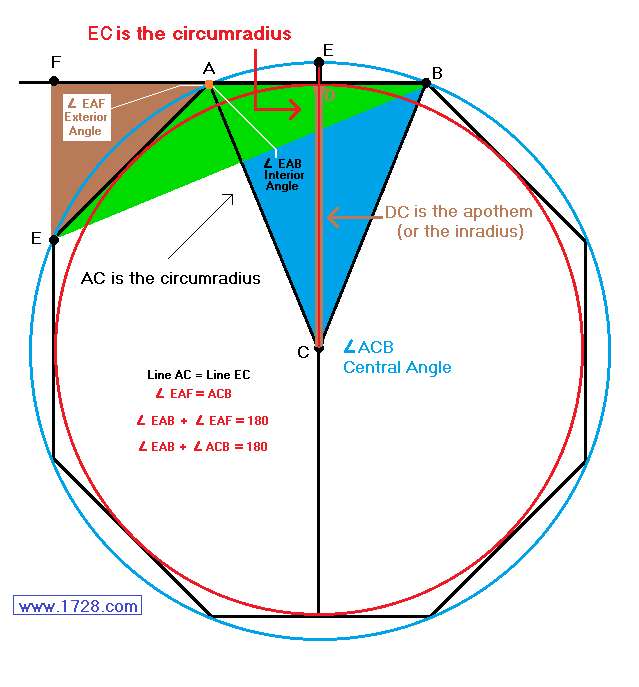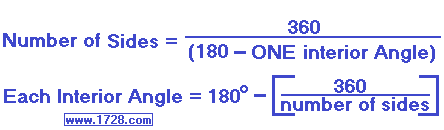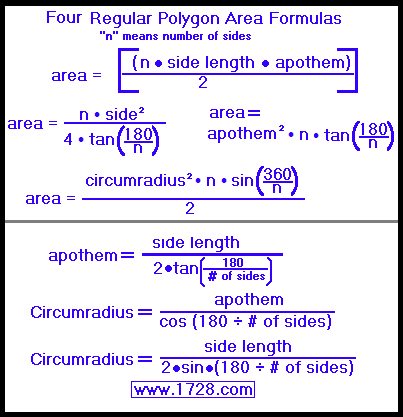Regular Polygon Calculator
Scroll down for Instructions and Definitions
To see a listing of the 22 polygons that have integer angles, click here.
Regular Polygons

This calculator works only for regular polygons - those polygons which have ALL sides equal & ALL interior angles equal.
The figure below shows a regular octagon.
The outer circle surrounding it is called a circumscribed circle (or circumcircle) and the inner circle which is surrounded by the octagon is called the inscribed circle (or incircle).
Angle EAB is one of the 8 interior angles.
Angle EAF is one of the 8 exterior angles and equals central angle ACB.
Line AC (which also equals Line EC) is called the circumradius (the radius of the outer, circumscribed circle).
Line DC is called the inradius or the apothem (the radius of the inscribed circle).
To see a list of the 22 polygons that have integer angles, click here.

Input data in the first 2 boxes on the right, then click 'Calculate'.Sum of Interior Angles = (Number of Sides -2) • 180 degrees

Number of Sides = (Sum of Interior Angles ÷ 180) + 2Each Exterior Angle = Each Central Angle = (360 degrees) ÷ (Number of Sides)Polygon Names
 Sides Name Sides Name Sides Name 3 triangle 9 nonagon 15 pentadecagon 4 quadrilateral 10 decagon 16 hexadecagon 5 pentagon 11 hendecagon 17 heptadecagon 6 hexagon 12 dodecagon 18 octadecagon 7 heptagon 13 tridecagon 19 enneadecagon 8 octagon 14 tetradecagon 20 icosagon

Significant Figures >>>
The default setting is for 5 significant figures but you can change that by inputting another number in the box above.

Answers are displayed in scientific notation and for easier readability, numbers between .001 and 1,000 will be displayed in standard format (with the same number of significant figures.)
The answers should display properly but there are a few browsers that will show no output whatsoever. If so, enter a zero in the box above. This eliminates all formatting but it is better than seeing no output at all.